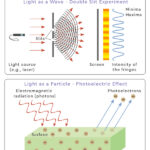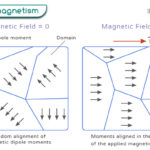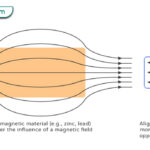Home / Physics / Buoyancy

# Buoyancy

## What is Buoyancy

When an object is immersed in a fluid, wholly or partially, the fluid exerts an upward force opposite its weight. This phenomenon is known as buoyancy, and the upward thrust is known as the buoyant force. A characteristic of buoyancy is that it determines whether an object will float or sink.

Who discovered buoyancy?

Greek mathematician Archimedes around 250 BC, discovered the law of buoyancy, and hence, it is today known as Archimedes’ principle.

## Buoyancy and Archimedes Principle

The Archimedes principle follows the law of buoyancy. According to this principle, when an object is immersed in a fluid, partially or wholly, it displaces the fluid. The weight lost by the object is equal to the weight of an equal volume of the displaced fluid.

## Buoyant Force: How does Buoyancy Work

When a solid object is immersed in a fluid, it experiences pressure in all directions, known as fluid pressure (Pascal’s principle). The fluid can be air or water. If the fluid is water, then it is known as hydrostatic pressure. The pressure increases with the depth of the fluid. Therefore, the pressure at the top of the object is less than that at the bottom. This pressure difference causes an upward force, known as the buoyant force. It is a contact force and opposite in direction to the weight of the object.

## Types of Buoyancy

There are three types of buoyancy.

### 1. Positive Buoyancy

When the weight of the fluid displaced by the object is more than the object’s weight, then the phenomenon is known as positive buoyancy. In this case, the object will float on the surface of the fluid.

### 2. Negative Buoyancy

When the weight of the fluid displaced is less than the object’s weight, it is called negative buoyancy. In this case, the object will be suspended in the fluid.

### 3. Neutral Buoyancy

When the weight of the fluid displaced is equal to the object’s weight, it is called neutral buoyancy. In this case, the object will sink.

## Buoyancy Examples

Here are some examples of the buoyant force in everyday life.

• Boat sailing on the river
• Iceberg floating on water
• A person with a life vest floating on water
• Ship floating on the ocean
• Helium balloon rising in the air

## Buoyant Force Equation: Law of Buoyancy

The buoyancy equation can be found by determining the displaced fluid’s weight and using the force balance equation.

### How to find Buoyant Force

Suppose an object of volume V is immersed in a fluid of density ρ. The object will displace an equal volume of the fluid. The mass of the liquid displaced is,

M = r x V

The weight of the displaced liquid is,

W = M x g

Or, W = r x V x g

According to Archimedes’ principle,

Loss of the object’s weight = Weight of the displaced liquid

This loss of weight is the thrust or buoyant force (Fb). Therefore,

Buoyant Force Formula: Fb = r X g X V

Unit of Buoyant Force: Newton or N

This equation is also called the law of buoyancy. It gives a relationship between buoyancy and density. As can be seen, the buoyant force is proportional to the density. The factors that affect buoyancy are:

• The density of the fluid
• The volume of the fluid displaced
• Acceleration due to gravity

The buoyant force is responsible for objects to float. The point on the object where it is applied is called the center of buoyancy.

Water Buoyancy: Water applies an upward thrust on objects that are immersed in it. The density of water is 997 kg/m3. Therefore, the buoyant force of water is.

Fb = 997 kg/m3 x 9.81 m/s2 x V

Or, Fb = 9781 kg/m2s2 x V

The density of saltwater is even higher at 1024 kg/m3, which explains why it is easier to float.

Air Buoyancy: Like water, air also exerts an upward thrust on objects that fall through or suspended in it. The density of air is 1.225 kg/m3.

## Applications of Buoyancy

Here are some real-life applications of buoyancy.

• To measure the volume and density of objects
• To measure the relative densities of liquids using a hydrometer
• To control the position and depth of a submarine by filling its ballast tank with water
• To control the position and height of a hot air balloon by varying the quantity of the hot air inside
• To enable fish to maneuver through water by filling its bladder with gas

Article was last reviewed on Friday, February 3, 2023

### Related articlesWave-Particle DualityPhotoelectric EffectFerromagnetismDiamagnetism

### One response to “Buoyancy”

1. Anagha g manoj says:

Very useful to me sir